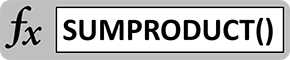# SUMPRODUCT Fundamentals – Multiply Without Adding ClutterNot every function has to be complicated in order to save time and clean up cluttered worksheets. SUMPRODUCT is a basic function that combines the uses of PRODUCT and SUM (brush up on the SUM formula here) to save space and improve readability of your models and dashboards. This quick tutorial will show you how it works…

## SUMPRODUCT Basics

The syntax of SUMPRODUCT is as follows:

`=SUMPRODUCT(array1,[array2],…)`

It takes any number of arrays, multiplies the corresponding cells, and then adds up the outcomes. The arrays have to be the same size for the math to work. Take a look at this quick example to see how SUMPRODUCT works, compared with the basic PRODUCT and SUM functions.

## SUMPRODUCT Example

In the example above, Array 1 (B2:C6) and Array 2 (E2:F6) are just sets of numbers. Using the PRODUCT function, we can multiply the cells together individually and it would look like the PRODUCT table (H2:I6). An example of the formula is in cell I2:

`=PRODUCT(C2,F2)`

It multiplies 6 by 16 and calculates to 96.

To get the total of these products, we have to sum them. We can sum the columns, as in H9 and H10:

`=SUM(H2:H6)`
`=SUM(I2:I6)`

Or we can sum the entire array, as in H11:

`=SUM(H2:I6)`

SUMPRODUCT lets us save time, economize on space, and get the same result. SUMPRODUCT can multiply two columns together and add them up, as in H12 and I12:

`=SUMPRODUCT(B2:B6,E2:E6)`
`=SUMPRODUCT(C2:C6,F2:F6)`

Finally, with its crowning achievement, SUMPRODUCT can multiply two arrays together pair-wise, and then add the results, as in cell H14:

`=SUMPRODUCT(B2:C6,E2:F6)`

By doing this, SUMPRODUCT can immediately get you the answer you want without all the clutter of the PRODUCT table and the separate SUM formulas. In a crowded spreadsheet, it’s a lifesaver, and you’ll find yourself using it again and again. Check out other functions in the SUM family, including the SUM function and SUMIF function!